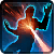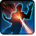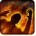# Heat BeamConditions

## Related effects, buffs and debuffs

Please click on an effect below to view its details.

• [hidden] [does not expire]Effect #1

 Slot: Debuff Duration: passive Tick rate: 1s # occurrences: 0 Conditions:
• On Apply

Perform the following actions:

• Play appearance epp . npc . droid . acklay_droid_dual . heat_beam . channel_tick, dependent on calling effect
• When effect ticks
• When effect is applied

Only when the following conditions are met:

• If difficulty is any of 8-man SM, 16-man SM, 16-man HM

Perform the following actions:

• Spell Damage
- Unknown (609) = (bool) false
- Damage Type = (int) 3
- Slot = (int) 1
- Spell Type = (int) 2
- Level Cap = (int) 0
- Amount Max = (float) 0
- Amount Min = (float) 0
- Amount Percent = (float) 0
- Threat Percent = (float) 0
- Standard Health Percent Max = (float) 0.03
- Standard Health Percent Min = (float) 0.02
- Amount Modifier Percent = (float) 0
- Coefficient = (float) 0
- Health Steal Percentage = (float) 0
• When effect ticks
• When effect is applied

Only when the following conditions are met:

• If difficulty is set to 8-man HM

Perform the following actions:

• Spell Damage
- Unknown (609) = (bool) false
- Damage Type = (int) 3
- Slot = (int) 1
- Spell Type = (int) 2
- Level Cap = (int) 0
- Amount Max = (float) 0
- Amount Min = (float) 0
- Amount Percent = (float) 0
- Threat Percent = (float) 0
- Standard Health Percent Max = (float) 0.04
- Standard Health Percent Min = (float) 0.03
- Amount Modifier Percent = (float) 0
- Coefficient = (float) 0
- Health Steal Percentage = (float) 0
• Just before this effect is removed

Perform the following actions:

• Play appearance epp . npc . droid . battle_droid . heat_beam . channel_end, dependent on calling effect
• When effect ticks

Perform the following actions:

• Add effect #2 to TARGET from TARGET, Combat Math Only
• [10s]Burning
Periodic elemental damage.

 Slot: Debuff Duration: 10s Tick rate: 1s # occurrences: 1 Tags: 15131558357926621105 Conditions: Can only by called by other effects
• When effect is applied

Perform the following actions:

• Play appearance epp . npc . droid . acklay_droid_dual . heat_beam . channel_tick, dependent on calling effect
• When effect ticks

Only when the following conditions are met:

• If difficulty is set to 8-man SM

Perform the following actions:

• Spell Damage
- Unknown (609) = (bool) false
- Damage Type = (int) 3
- Slot = (int) 1
- Spell Type = (int) 2
- Level Cap = (int) 0
- Amount Max = (float) 0
- Amount Min = (float) 0
- Amount Percent = (float) 0
- Threat Percent = (float) 0
- Standard Health Percent Max = (float) 0.03
- Standard Health Percent Min = (float) 0.02
- Amount Modifier Percent = (float) 0
- Coefficient = (float) 0
- Health Steal Percentage = (float) 0
• When effect ticks

Only when the following conditions are met:

• If difficulty is set to 8-man HM

Perform the following actions:

• Spell Damage
- Unknown (609) = (bool) false
- Damage Type = (int) 3
- Slot = (int) 1
- Spell Type = (int) 2
- Level Cap = (int) 0
- Amount Max = (float) 0
- Amount Min = (float) 0
- Amount Percent = (float) 0
- Threat Percent = (float) 0
- Standard Health Percent Max = (float) 0.05
- Standard Health Percent Min = (float) 0.04
- Amount Modifier Percent = (float) 0
- Coefficient = (float) 0
- Health Steal Percentage = (float) 0